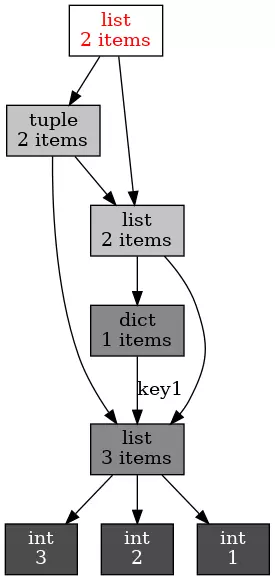# 深入python内存管理

python内存管理是由私有堆空间管理的，所有的python对象和数据结构都存储在私有堆空间中。程序员没有访问堆的权限，只有解释器才能操作。为python的堆空间分配内存的是python的内存管理模块进行的，核心api会提供一些访问该模块的方法供程序员使用。python自有的垃圾回收机制回收并释放没有被使用的内存供别的程序使用。

### 对象的内存使用

 ``````1 `````` ``````a = 1 ``````

 ``````1 2 3 4 5 `````` ``````>>> a = 1 >>> id(a) 140035503539424 # 内存地址的十进制表示 >>> hex(id(a)) '0x7f5c8e71c4e0' # 内存地址的十六进制表示 ``````

 ``````1 2 3 `````` ``````>>> b = 1 >>> id(b) 140035503539424 # 等于上面id(a)的值 ``````

 `````` 1 2 3 4 5 6 7 8 9 10 11 12 13 14 15 16 17 18 19 20 21 22 23 24 25 `````` ``````a = 1 b = 1 print(a is b) True a = "good" b = "good" print(a is b) True a = "very good morning" b = "very good morning" print(a is b) True # 原文在此处就是False，但是我的为True，通过查资料发现是python版本原因 # Python2.3简单整数缓存范围是(-1,100)，Python2.5.4以后简单整数缓存范围至少是(-5,256)。所有的短字符也都在缓存区。 a = "为了校验两个引用指向同一个对象，我们可以用“is”来判断。is用于判断两个引用所指的对象是否相同。" b = "为了校验两个引用指向同一个对象，我们可以用“is”来判断。is用于判断两个引用所指的对象是否相同。" print(a is b) False # 增加了字符串的长度，结果也是False a = [] b = [] print(a is b) False ``````

 ``````1 2 3 4 5 6 7 8 `````` ``````from sys import getrefcount a = [1, 2, 3] print(getrefcount(a)) # 2 b = a print(getrefcount(b)) # 3 ``````

### 对象引用对象

python的一个容器对象（container），比如列表字典等，可以包含多个对象。实际上，容器对象中包含的并不是对象本身，而是指向各个元素对象的引用。

 ``````1 2 3 4 5 6 7 8 9 `````` ``````class from_obj(object): def __init__(self, to_obj): self.to_obj = to_obj b = [1,2,3] a = from_obj(b) print(id(a.to_obj)) print(id(b)) # 140035473779144 # 140035473779144 ``````

 ``````1 2 3 4 5 6 7 `````` ``````from sys import getrefcount a = [1, 2, 3] print(getrefcount(a)) b = [a, a] print(getrefcount(a)) # 2 # 4 ``````

``````pip install objgraph
``````

 ``````1 2 3 4 5 6 7 `````` ``````sudo pacman -S xdot或者 sudo apt install xdot，sudo yun install xdot x = [1, 2, 3] y = [x, dict(key1=x)] z = [y, (x, y)] import objgraph objgraph.show_refs([z], filename='ref_topo.png') ````````````1 2 3 4 5 `````` ``````>>> a = [] >>> b = [a] >>> a.append(b) >>> a [[[...]]] ``````

 ``````1 2 3 4 `````` ``````>>> a = [] >>> a.append(a) >>> print(getrefcount(a)) 3 ``````

### 引用减少

 ``````1 2 3 4 5 6 7 `````` ``````>>> a = [1, 2, 3] >>> b = a >>> print(getrefcount(b)) 3 >>> del a >>> print(getrefcount(b)) 2 ``````

del也可以删除容器中的元素，比如：

 `````` 1 2 3 4 5 6 7 8 9 10 11 `````` ``````>>> a = [1,2,3] >>> del a >>> print(a) [2, 3] >>> b = {"q": 1, "w":2} >>> b {'q': 1, 'w': 2} >>> del b["q"] >>> b {'w': 2} ``````

 `````` 1 2 3 4 5 6 7 8 9 10 `````` ``````>>> from sys import getrefcount ... ... a = [1, 2, 3] ... b = a ... print(getrefcount(b)) ... ... a = 1 ... print(getrefcount(b)) 3 2 ``````

### 垃圾回收

 ``````1 2 `````` ``````a = [1,2,3] del a ``````

`del a`之后已经没有任何引用指向[1,2,3]了，用户不可能通过任何方式接触或者动用这个对象，这个对象如果继续待在内存里，就成了不健康的数据。当python的垃圾回收机制启动的时候，python扫描到这个引用为0的对象，就会将它所占据的内存清空。

 ``````1 2 3 `````` ``````>>> import gc >>> gc.get_threshold() (700, 10, 10) ``````

### 分代回收

python同时使用了分代（generation）回收的策略。这一策略的基本假设是，存活时间越久的对象，越不可能在后面的程序中变成垃圾。我们的程序往往会产生大量的对象，许多对象很快产生和消失，但也有长期存在被使用的对象，出于信任和效率，对于这样一些对象，我们相信他的用处，所以减少在垃圾回收中扫描他们的频率。

python将所有的对象分为0,1,2三代，所有新建的对象都是0代对象，当某一代对象经历过垃圾回收之后，依然存活，那就归入到下一代中，垃圾回收启动时，一定会扫描所有的0代对象。如果0代对象经历过一定次数的垃圾回收，那么就启动对0待和1代的扫描清理，当1代也经历了一定数量的垃圾回收，那就启动对0,1,2，即所有的对象进行扫描。

 ``````1 2 `````` ``````import gc gc.set_threshold(700, 10, 5) ``````

### 孤立的引用环

 ``````1 2 3 4 5 `````` ``````a = [] b = [a] a.append(b) del a del b ``````

### 总结

python作为一种动态类型的语言，其对象和引用分离，这与面向过程的编程语言有很大的区别。为了有效的释放内存，python内置了垃圾回收的支持。python采用了一种相对简单的垃圾回收机制，即引用计数，并因此需要解决孤立引用环的问题。Python与其它语言既有共通性，又有特别的地方。对该内存管理机制的理解，是提高Python性能的重要一步。